## Example Questions

← Previous 1

### Example Question #1 : How To Find A Solution Set

|2x – 25| – 3 = 7. There are two solutions to this problem. What is the sum of those solutions?

25

17

7.5

10

25

Explanation:

First, simplify the equation so the absolute value is all that remains on the left side of the equation:

|2x – 25| = 10

Now create two equalities, one for 10 and one for –10.

2x – 25 = 10        and        2x – 25 = –10

2x = 35               and        2x = 15

x = 17.5              and          x = 7.5

The two solutions are 7.5 and 17.5.  17.5 + 7.5 = 25

### Example Question #2 : How To Find A Solution Set

Find the roots of the equation x+ 5x + 6.

1, 4

2, 3

–2, –3

–2, 3

2, –3

–2, –3

Explanation:

Factoring gives us (x + 2)(x + 3). This yields x = –2, –3.

### Example Question #3 : How To Find A Solution Set

When you divide a number by 3 and then add 2, the result is the same as when you multiply the same number by 2 then subtract 23. What is the number?

9

2

15

7

3

15

Explanation:

You set up the equation and you get: (x/3) + 2 = 2x – 23.

Add 23 to both sides: (x/3) + 25 = 2x

Multiply both sides by 3: x + 75 = 6x

Subtract x from both sides: 75 = 5x

Divide by 5 and get = 15

### Example Question #4 : How To Find A Solution Set

Find the sum of the solutions to the equation:

2x – 2x  – 2 = 1 – x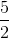Explanation:

First, we need to get everything on one side so that the equation equals zero.

2x- 2x -2 = 1-x

We need to add x to the left, and then subtract 1.

2x- 2x -2 +x - 1 = 0

2x- x - 3 = 0

Now we need to factor the binomial. In order to do this, we need to multiply the outer two coefficients, which will give us 2(-3) = -6. We need to find two numbers that will mutiply to give us -6. We also need these two numers to equal -1 when we add them, because -1 is the coefficient of the x term.

If we use +2 and -3, then these two numbers will multiply to give us -6 and add to give us -1. Now we can rewrite the equation as follows:

2x- x - 3 = 2x+ 2x - 3x - 3 = 0

2x+ 2x - 3x - 3 = 0

Now we can group the first two terms and the last two terms. We can then factor the first two terms and the last two terms.

2x(x+1) -3(x+1) = 0

(2x-3)(x+1) = 0

This means that either 2x - 3 = 0, or x + 1 = 0. So the values of x that solve the equation are 3/2 and -1.

The question asks us for the sum of the solutions, so we must add 3/2 and -1, which would give us 1/2.

### Example Question #5 : How To Find A Solution Set

If 3y = 2x – 7, then which of the following statements is correct?

y is greater

x is greater

not enough information given

they are equal

not enough information given

Explanation:

If we set one variable to the other we would get y = (2x – 7)/3 or x = (3y + 7)/2, but we aren't given any clues to what the values of x and y are and we can assume they could be any number. If x = 7/2, then y = 0. If y = -7/3, then x = 0. Let's try some other numbers. If y = –10, then x = –37/2. So for the first two examples, x is greater than y. In the last example, y is greater than x. We need more information to determine whether x or y is greater.  The correct answer is not enough information given.

### Example Question #6 : How To Find A Solution Set

|10  2| – |1 – 9| = ?

8

0

2

16

0

Explanation:

When taking the absolute value we realize that both absolute value operations yield 8, which gives us a difference of 0.

### Example Question #7 : How To Find A Solution Set

When you multiply a number by 5 and then subtract 23, the result is the same as when you multiplied the same number by 3 then added 3. What is the number?

7

13

10

5

6

13

Explanation:

You set up the equation 5x – 23 = 3x + 3, then solve for x, giving you 13.

### Example Question #8 : How To Find A Solution Set

What is the product of the two values ofthat satisfy the following equation?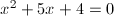Explanation:

First, solve for the values of x by factoring.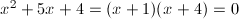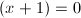or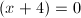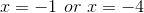Then, multiply the solutions to obtain the product.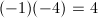### Example Question #9 : How To Find A Solution Set

Solve for y: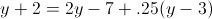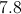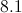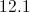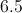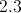Explanation:

Collecting terms leaves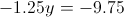And dividing by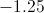yields### Example Question #10 : How To Find A Solution Set

Solve for x.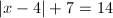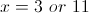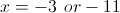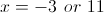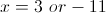Explanation: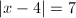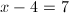or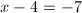← Previous 1